Refer to our Texas Go Math Grade 1 Answer Key Pdf to score good marks in the exams. Test yourself by practicing the problems from Texas Go Math Grade 1 Lesson 16.3 Answer Key Fourths.

Explore

Use what you know about halves. Draw to solve. Write how many.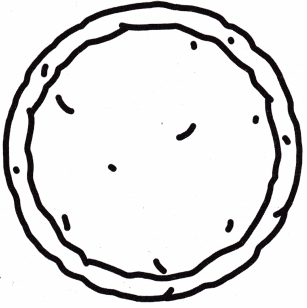There are ___________ equal shares.
Answer: There are Four equal shares.

FOR THE TEACHER • Read the following problem. Two friends will share a pizza. Then two more friends come, Now four friends will share the pizza. How can the pizza be cut so each friend gets an equal share? How many equal shares are there?
Answer: There are Four equal shares.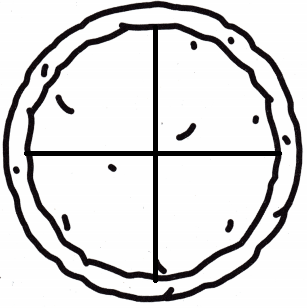Explanation:
To share the pizza with four friends it have to be divided into four equal parts.
so, The pizza have to be cut into four so each friend gets an equal share.

Math Talk
Mathematical Processes

How did you decide how to cut the pizza?
Explanation:
To share the pizza with four friends it have to be divided into four equal parts.
so, The pizza have to be cut into four so each friend gets an equal share.

Model and Draw

The 4 equal shares make 1 whole.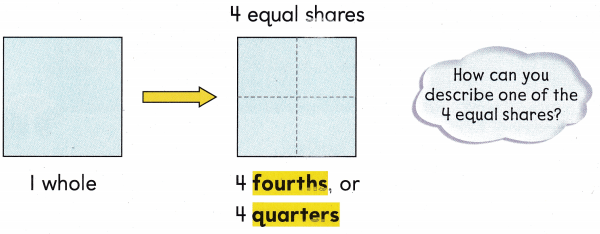Share and Show

Draw lines to show fourths.

Question 1.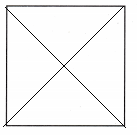Question 2.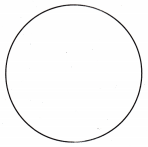Question 3.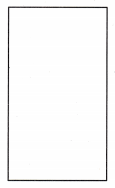Question 4.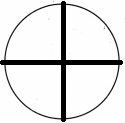Question 5.Question 6.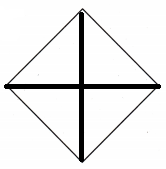Problem Solving

Name the parts. Write fourths or not fourths.

Question 7.Question 8.Question 9.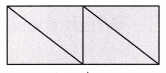Question 10.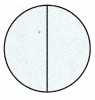Question 11.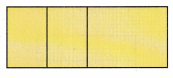Question 12.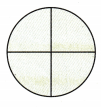Question 13.
H.O.T. Circle the shape that shows quarters.Question 14.
H.O.T. Multi-Step Draw three different ways to show fourths.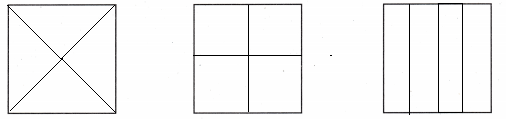Question 15.
Representations Mick wants to build a toy box. It will have 4- equal parts. Which shows fourths?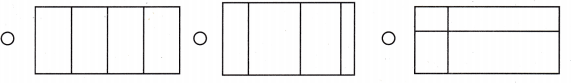Question 16.
Which shape does not show fourths.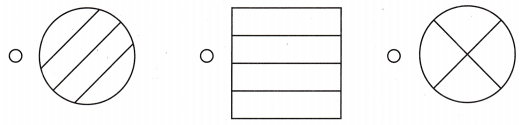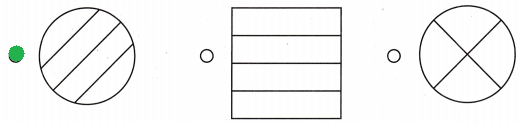Question 17.
Multi-Step Billy will share his cake with 3 friends. Each person will get a fourth. He hands out I piece. He eats I piece. How many pieces does he have left?
(A) 2
(B) 4
(C) 1
Explanation:
Billy cuts the cake into four equal shares as he have to share it with 3 friend and for himself.
As Billy eats his share and hands out one piece his is now left with two more pieces.
1+1=2
4-2=2

Question 18.
Texas Test Prep Which shows fourths?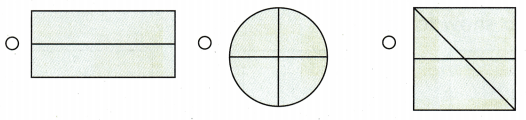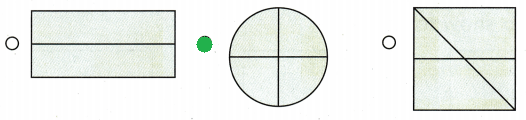TAKE HOME ACTIVITY • Draw a circle on a piece of paper. Ask your child to draw lines to show fourths.### Texas Go Math Grade 1 Lesson 16.3 Homework and Practice Answer Key

Color a fourth of the shape.

Question 1.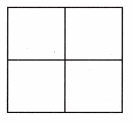Question 2.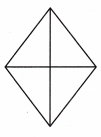Question 3.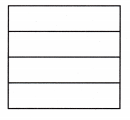Color a quarter of the shape.

Question 4.Question 5.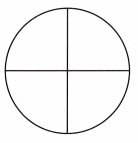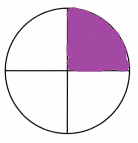Question 6.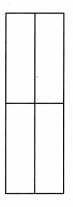Problem Solving

Draw three different ways to show fourths.

Question 7.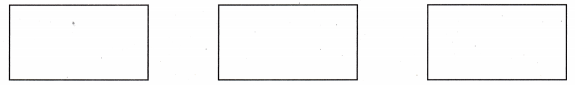Lesson Check

Question 8.
Tina wants to paint a wall. The wall will have 4 equal parts. Which shows fourths?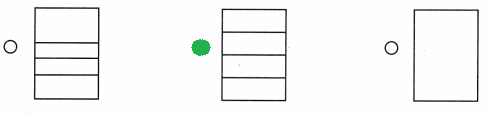Question 9.
Which shows quarters?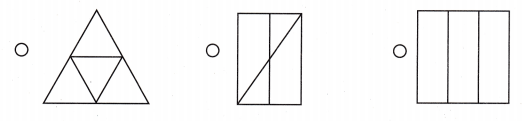Question 10.
Multi-Step Isabelle shares a pizza with her mother, her brother, and her father. Each person will get a fourth. She hands out 2 pieces. She eats I piece. How many pieces does she have left?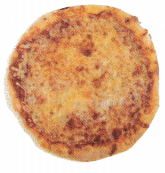(A) 0
(B) 3
(C) 1